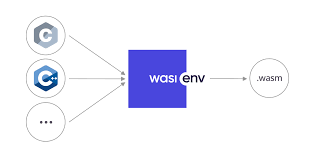# WebAssembly with C++## Environment Setup​

To compile this demo, you must install the following:

### C++​

Go to C and C++ Installation and follow the instructions.

### Wasmer​

Wasmer is an open-source runtime for executing WebAssembly on the Server.

#### Wasienv​

Wasienv is a tool to compile different programming languages to WebAssembly, so you can run your programs on the Browser, or in the Server.

### Wasmtime​

You will find wasmtime at wasmtime.dev

## C++ Code Snippet​

We will create a Simple C++ Program that will return us the fibonacci sequence of an Integer Input.

Create a folder "C++-to-WASM":

``mkdir C++-to-WASMcd C++-to-WASM``

Create a file named `FibonacciSequence.cpp`:

``// Simple Program to calculate Fibonacci Sequence of an integer input#include<iostream>using namespace std;int FibonacciSequence(int num) {    if(num <= 1) {        return num ;    }    return FibonacciSequence(num-1) + FibonacciSequence(num-2);}int main(){    cout << "Enter the Number" << endl;    int n ;    cin  >> n ;        cout << "Fibonacci Sequence term at " << n << "  " << "is " << FibonacciSequence(n) << endl;}``

## Compiling the C++ Code​

1. Compiling Using `g++`
``g++ FibonacciSequence.cpp``1. Compile to WASM Binary using the following Command:

Since the Code has been written in C++, we need to figure out a way to generate a WebAssembly Binary. That's why we will be using `wasienv` in order to generate a `.wasm` binary from this `cpp` file.

When you have your `cpp` file created, you can execute `wasic++`

`` wasic++ FibonacciSequence.cpp -o FibonacciBinary.wasm``

Note that while executing this command, it might generate some warnings but you can ignore them.

1. Now, you will have a new `FibonacciBinary.wasm` file ready in your Directory

2. Executing it using WASM Runtime

``wasmtime FibonacciBinary.wasm``